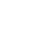# Use Selectors to Calculate Derived Data Based on State Stored within a Recoil Atom

Published a year ago
Updated 3 months ago

Recoil allows us to use atoms in order to store pieces of state. More often than not in our apps we need to use data that derives from our application state (for instance to multiply height and width stored within a state to calculate area of an element).

Luckily Recoil provides us with a powerful tool to automatically re-calculate derived state value whenever an piece of state changes - selectors.

A selector is a pure function that accepts atoms or other selectors as input. When these upstream atoms or selectors are updated, the selector function will be re-evaluated.

In this quick lesson we're going to learn how to use a selector in order to automatically calculate a square of a number whenever a value stored within an atom is going to change

Tomasz Łakomy: [0:00] We've added one more component to our app and that is the Square component. Here, what we would like to do is to get the value stored within the numState and calculate the square of it. For 4, it's going to be 16, for 5, it's going to be 25, and so on.

[0:13] In other words, we would like to be able to make some calculation based on the value stored within this atom. Whenever this atom is going to be updated by either the counter component or some other component in our app, we would like the square value to be recalculated. In order to do that, we are going to use Recoil selectors.

[0:29] First up, import selector from "recoil". In Recoil, a selector is a pure function that accepts atoms or some other selectors as input. What's going to happen is that a selector is going to calculate a derived state.

[0:44] In that case, you can think of derived state as the output of passing the state, in our case the numState, to a pure function that is going to modify the state in some way such as calculating a square of the number stored within the numState atom.

[0:57] To create a selector, let's do const squareState = selector. That is going to take an object as an argument. Similar to an atom, we have to specify a key. In that case, it's going to be key: "squareState". Secondly, we have to specify a get property. This get property is the function that it is to be computed. This function can access the value of atoms or some other selectors.

[1:20] First, let me create a function. We have to get the get argument that is passed to it. This get argument is a function that we can use to get values from atoms and other selectors. Here, I'm going to do return get(numState) **2. I'm going to square it as well so that our squareState is going to be always equal to a square of this numState. That is awesome.

[1:41] Let's use our brand-new selector. I'm going to scroll down over here. In the Square method, I'm going to do const squareNumber. I'm going to do useRecoilValue. I'm going to get the squareState. Instead of displaying that, I'm going to display the squareNumber.

[1:57] If I save that, our app is going to work as intended. Right now, if I click on Increment, the number is going to be equal to 4 and the square is going to be equal to 16.

[2:06] To recap, in Recoil, if you want to keep the track of core pieces of our state, we have to use atoms, but if you would like to calculate some other pieces of state based on those atoms, we have to use selectors.

[2:18] Selectors are really powerful because as we see in this example, whenever the numState is going to be changed by any component, the squareState is going to be recalculated automatically because it is based on this numState. This approach allows us to have a minimum number of atoms and only use the selectors to calculate derived data from those atoms.Nate Holloway
~ a year ago

`const squareState = (number) => number**2;`

`const squareNumber = squareState(useRecoilValue(numstate));`

Reuse: The selector can be used in all components.Jose Torres
~ a year ago

where do we should define the selector? In the same place we defined the atom or in other place?Eleonora Lester
~ a year ago

If we need to reuse that selector, how do we pass the atom to it if we move the selector to a separate file?